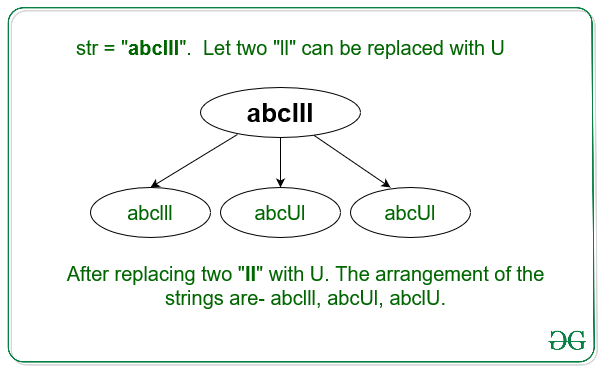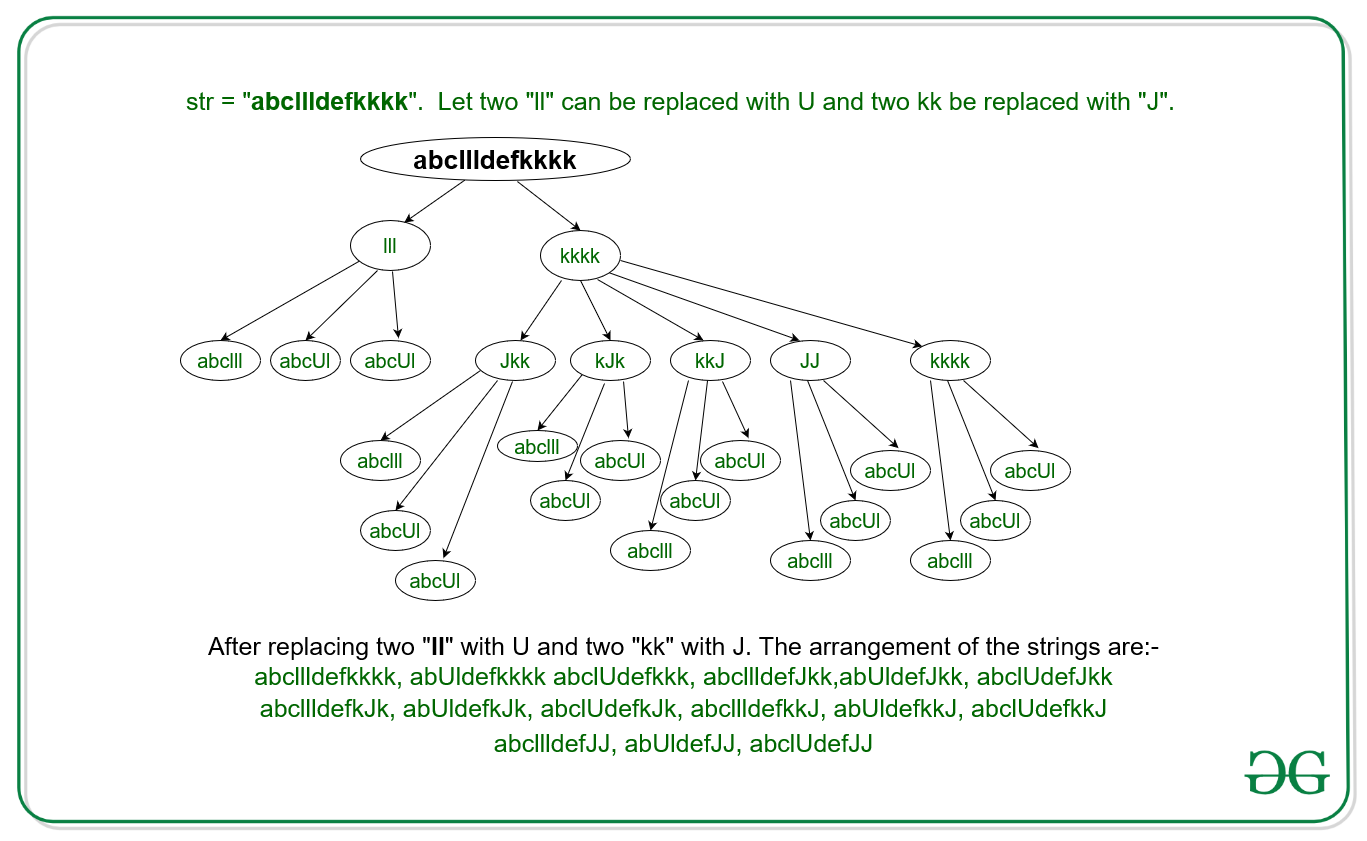Related Articles

# Count of strings possible by replacing two consecutive same character with new character

• Last Updated : 03 May, 2021

Given string str. The task is to count the number of all different strings possible if two consecutive same characters of the string can be replaced by one different character.
Examples

Input: str = “abclll”
Output:
Explanation:
There can be 3 different string including the original string as shown in the below figure:-Input: str = “abcllldefkkkk”
Output: 15
Explanation:
There can be 15 different string including the original string as shown in the below figure:-Approach:
Following properties were observed for replacing two equals characters at a time:

• If for string = “aaa” of length 3 we replace two “aa” with one character say “K” then the total number of different possible strings including the original string is:- Ka, aK, aaa. Therefore the number of different strings follows the property of Fibonacci Number of the length of the consecutive characters in the string.
• If for string = “aaadefyyyy” then the total number of possible different strings is equaled to the product of combinations of string “aaa” and “yyyy” by replacing the two consecutive characters at a time.

Hence from the above two observations, the count of different possible strings with N consecutive characters is given by N-th Fibonacci Number. Therefore, the total number of different possible strings for the given string str is equal to the product of count of different possible strings for every substring with all same character.
Following are the steps:

1. Count(say cnt) the number of consecutive characters which are same in the given string str.
2. To count the different possible strings for the count cnt, find the value of Fibonacci Sequence at cnt.
3. Repeat the above steps for all the consecutive characters in the given string str.
4. The total count of different possible strings is equaled to the product of all the value of Fibonacci Sequence obtained for every count of consecutive characters.

Below is the implementation of the above approach:

## C++

 `// C++ program to count the``// different possible string``// form by replacing two same``// characters with one``#include ``using` `namespace` `std;` `// Array to find the fibonacci``// sequence``int` `fib;` `// Function to find the``// fibonacci sequence``void` `computeFibonacci()``{``    ``fib = 1;``    ``fib = 1;``    ``for` `(``int` `i = 2; i < 100005; i++) {``        ``fib[i] = fib[i - 1] + fib[i - 2];``    ``}``}` `// Function to count all``// possible strings``int` `countString(string str)``{` `    ``// Initialize ans = 1``    ``int` `ans = 1;``    ``int` `cnt = 1;` `    ``for` `(``int` `i = 1; str[i]; i++) {` `        ``// If two consecutive``        ``// char are same``        ``// increase cnt``        ``if` `(str[i] == str[i - 1]) {``            ``cnt++;``        ``}` `        ``// Else multiply the``        ``// fib[cnt] to ans``        ``// and initialize ans``        ``// to 1``        ``else` `{``            ``ans = ans * fib[cnt];``            ``cnt = 1;``        ``}``    ``}``    ``//``    ``// If str = abcdeeee, then``    ``// for last "eeee" the``    ``// count munst be updated``    ``ans = ans * fib[cnt];` `    ``// Return the total count``    ``return` `ans;``}` `// Driver's Code``int` `main()``{``    ``string str = ``"abdllldefkkkk"``;` `    ``// Function to precompute``    ``// all the fibonacci number``    ``computeFibonacci();` `    ``// Function call to find``    ``// the count``    ``cout << countString(str);``    ``return` `0;``}`

## Java

 `// Java program to count the``// different possible string``// form by replacing two same``// characters with one``class` `GFG {``    ` `    ``// Array to find the fibonacci``    ``// sequence``    ``static` `int` `fib[] = ``new` `int``[``100005``];``    ` `    ``// Function to find the``    ``// fibonacci sequence``    ``static` `void` `computeFibonacci()``    ``{``        ``fib[``0``] = ``1``;``        ``fib[``1``] = ``1``;``        ``for` `(``int` `i = ``2``; i < ``100005``; i++) {``            ``fib[i] = fib[i - ``1``] + fib[i - ``2``];``        ``}``    ``}``    ` `    ``// Function to count all``    ``// possible strings``    ``static` `int` `countString(String str)``    ``{``    ` `        ``// Initialize ans = 1``        ``int` `ans = ``1``;``        ``int` `cnt = ``1``;``    ` `        ``for` `(``int` `i = ``1``; i

## Python3

 `# Python3 program to count the``# different possible string``# form by replacing two same``# characters with one` `# Array to find the fibonacci``# sequence``fib ``=` `[``0``]``*``100005``;` `# Function to find the``# fibonacci sequence``def` `computeFibonacci() :` `    ``fib[``0``] ``=` `1``;``    ``fib[``1``] ``=` `1``;``    ``for` `i ``in` `range``(``2``, ``100005``) :``        ``fib[i] ``=` `fib[i ``-` `1``] ``+` `fib[i ``-` `2``];` `# Function to count all``# possible strings``def` `countString(string) :` `    ``# Initialize ans = 1``    ``ans ``=` `1``;``    ``cnt ``=` `1``;` `    ``for` `i ``in` `range``(``1``, ``len``(string)) :` `        ``# If two consecutive``        ``# char are same``        ``# increase cnt``        ``if` `(string[i] ``=``=` `string[i ``-` `1``]) :``            ``cnt ``+``=` `1``;` `        ``# Else multiply the``        ``# fib[cnt] to ans``        ``# and initialize ans``        ``# to 1``        ``else` `:``            ``ans ``=` `ans ``*` `fib[cnt];``            ``cnt ``=` `1``;``            ` `    ``# If str = abcdeeee, then``    ``# for last "eeee" the``    ``# count munst be updated``    ``ans ``=` `ans ``*` `fib[cnt];` `    ``# Return the total count``    ``return` `ans;` `# Driver's Code``if` `__name__ ``=``=` `"__main__"` `:` `    ``string ``=` `"abdllldefkkkk"``;` `    ``# Function to precompute``    ``# all the fibonacci number``    ``computeFibonacci();` `    ``# Function call to find``    ``# the count``    ``print``(countString(string));``    ` `# This code is contributed by Yash_R`

## C#

 `// C# program to count the``// different possible string``// form by replacing two same``// characters with one``using` `System;` `class` `GFG {``    ` `    ``// Array to find the fibonacci``    ``// sequence``    ``static` `int` `[]fib = ``new` `int``;``    ` `    ``// Function to find the``    ``// fibonacci sequence``    ``static` `void` `computeFibonacci()``    ``{``        ``fib = 1;``        ``fib = 1;``        ``for` `(``int` `i = 2; i < 100005; i++) {``            ``fib[i] = fib[i - 1] + fib[i - 2];``        ``}``    ``}``    ` `    ``// Function to count all``    ``// possible strings``    ``static` `int` `countString(``string` `str)``    ``{``    ` `        ``// Initialize ans = 1``        ``int` `ans = 1;``        ``int` `cnt = 1;``    ` `        ``for` `(``int` `i = 1; i < str.Length; i++) {``    ` `            ``// If two consecutive``            ``// char are same``            ``// increase cnt``            ``if` `(str[i] == str[i - 1]) {``                ``cnt++;``            ``}``    ` `            ``// Else multiply the``            ``// fib[cnt] to ans``            ``// and initialize ans``            ``// to 1``            ``else` `{``                ``ans = ans * fib[cnt];``                ``cnt = 1;``            ``}``        ``}``         ` `        ``// If str = abcdeeee, then``        ``// for last "eeee" the``        ``// count munst be updated``        ``ans = ans * fib[cnt];``    ` `        ``// Return the total count``        ``return` `ans;``    ``}``    ` `    ``// Driver's Code``    ``public` `static` `void` `Main (``string``[] args)``    ``{``        ``string` `str = ``"abdllldefkkkk"``;``    ` `        ``// Function to precompute``        ``// all the fibonacci number``        ``computeFibonacci();``    ` `        ``// Function call to find``        ``// the count``        ``Console.WriteLine(countString(str));``    ``}``}` `// This code is contributed by AnkitRai01`

## Javascript

 ``
Output:
`15`

Time Complexity: O(N), where N is the length of the given string.My Personal Notes arrow_drop_up